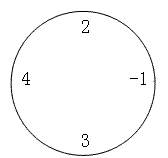编程知识 购物 网址 新闻 笑话 | 软件 日历 阅读 图书馆 China
 TxT小说阅读器 ↓语音阅读,小说下载,古典文学↓ 图片批量下载器 ↓批量下载图片,美女图库↓ 图片自动播放器 ↓图片自动播放器↓ 一键清除垃圾 ↓轻轻一点,清除系统垃圾↓vbs/VBScript DOS/BAT hta htc python perl 游戏相关 VBA 远程脚本 ColdFusion ruby专题 autoit seraphzone PowerShell linux shell Lua Golang Erlang 其它教程 CSS/HTML/Xhtml html5 CSS XML/XSLT Dreamweaver教程 经验交流
 站长资讯 .NET新手 ASP.NET C# WinForm Silverlight WCF CLR WPF XNA VisualStudio ASP.NET-MVC .NET控件开发 EntityFramework WinRT-Metro Java C++ PHP Delphi Python Ruby C语言 Erlang Go Swift Scala R语言 Verilog 其它语言 架构设计 面向对象 设计模式 领域驱动 Html-Css JavaScript jQuery HTML5 SharePoint GIS技术 SAP OracleERP DynamicsCRM K2 BPM 信息安全 企业信息 Android开发 iOS开发 WindowsPhone WindowsMobile 其他手机 敏捷开发 项目管理 软件工程 SQLServer Oracle MySQL NoSQL 其它数据库 Windows7 WindowsServer Linux
 -> C++ -> 洛谷P1043 数字游戏 -> 正文阅读

### [C++]洛谷P1043 数字游戏

 题目描述丁丁最近沉迷于一个数字游戏之中。这个游戏看似简单，但丁丁在研究了许多天之后却发觉原来在简单的规则下想要赢得这个游戏并不那么容易。游戏是这样的，在你面前有一圈整数（一共n个），你要按顺序将其分为m个部分，各部分内的数字相加，相加所得的m个结果对10取模后再相乘，最终得到一个数k。游戏的要求是使你所得的k最大或者最小。例如，对于下面这圈数字（n=4，m=2）：要求最小值时，((2-1) mod 10)×((4+3) mod 10)=1×7=7，要求最大值时，为((2+4+3) mod 10)×(-1 mod 10)=9×9=81。特别值得注意的是，无论是负数还是正数，对10取模的结果均为非负值。丁丁请你编写程序帮他赢得这个游戏。输入输出格式输入格式：输入文件第一行有两个整数，n（1≤n≤50）和m（1≤m≤9）。以下n行每行有个整数，其绝对值不大于104，按顺序给出圈中的数字，首尾相接。输出格式：输出文件有两行，各包含一个非负整数。第一行是你程序得到的最小值，第二行是最大值。输入输出样例输入样例#1： 复制 ```4 2 4 3 -1 2 ```输出样例#1： 复制 ```7 81 ```\$DpMin[i][j][k]\$表示\$i\$到\$j\$中切了\$k\$次的最小值\$DpMax\$表示最大值转移的时候枚举断点左右两边相乘 ```#include #include #include using namespace std; const int MAXN=2001; const int INF=0x7fffff; inline char nc() { static char buf[MAXN],*p1=buf,*p2=buf; return p1==p2&&(p2=(p1=buf)+fread(buf,1,MAXN,stdin),p1==p2)?EOF:*p1++; } inline int read() { char c=nc();int x=0,f=1; while(c<'0'||c>'9'){if(c=='-')f=-1;c=nc();} while(c>='0'&&c<='9'){x=x*10+c-'0';c=nc();} return x*f; } int DpMin; int DpMax; int a[MAXN]; int Query(int a) { return ((a%10)+10)%10; } int main() { #ifdef WIN32 freopen("a.in","r",stdin); #else #endif int n=read(),m=read(); for(int i=1;i<=n;i++) a[i]=read(),a[i+n]=a[i]; for(int i=1;i<=2*n;i++) a[i]+=a[i-1]; for(int l=1;l<=2*n;l++) for(int r=l;r<=2*n;r++) DpMin[l][r]=DpMax[l][r]=Query(a[r]-a[l-1]); for(int i=2;i<=m;i++) for(int l=1;l<=2*n;l++) for(int r=l+i-1;r<=2*n;r++) DpMin[l][r][i]=INF; for(int i=2;i<=m;i++)//已经切了k次 for(int l=1;l<=2*n;l++) for(int r=l+i-1;r<=2*n;r++) for(int k=l+i-2;k
 上一篇文章      下一篇文章      查看所有文章
 加:2017-12-05 23:23:29  更:2017-12-05 23:23:33
 360图书馆 软件开发资料 购物精选 新闻资讯 Chinese Culture 龙发科技 开发 中国文化 阅读网 日历 万年历 2020年8日历2020-8-15 1:53:39
 多播视频美女直播 ↓电视,电影,美女直播,迅雷资源↓ TxT小说阅读器 ↓语音阅读,小说下载,古典文学↓ 一键清除垃圾 ↓轻轻一点,清除系统垃圾↓ 图片批量下载器 ↓批量下载图片,美女图库↓
 网站联系: qq:121756557 email:121756557@qq.com  编程知识